## Python —— 从零到远方 第一章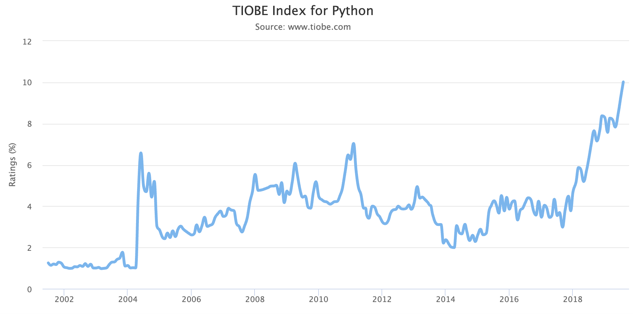1 PythonTIOBE指数

Python确实是一门神奇的计算机语言，从TIOBE编程语言指数上看，Python近年来上涨的非常快，已经稳居第三名，指数超过第四名——曾经无比神奇的C++4%之多。前两名是Java和C语言，一个是几乎所有安卓手机都在用的Java语言，另一个是几乎所有嵌入式系统和操作系统都在用的C语言。

Python可能是对于非计算机专业的学生、学者和爱好者非常友好的计算机语言了，上手难度在编程语言中是非常低的，而能够实现的功能和算法却非常专业和高效。Python就像是一辆调教良好的自动挡汽车，好开又省油。这并不是说计算机专业的学生就不要学Python了，恰恰相反，试问有多少人考了C1驾照还开手动挡汽车的？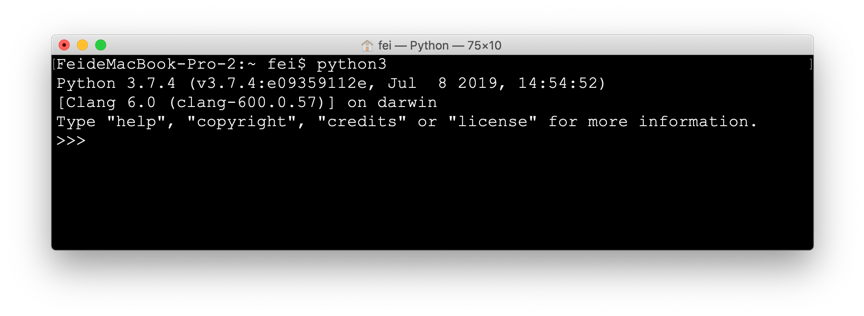2 Python Shell界面 (我的Terminal改成了黑框框)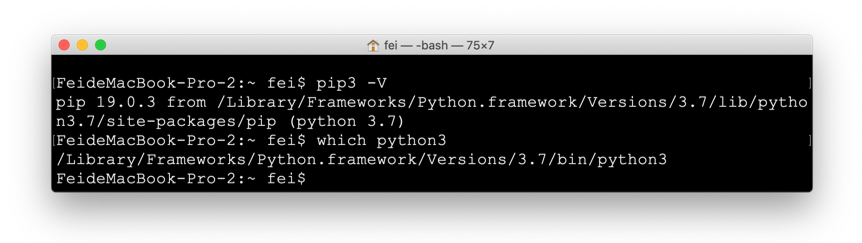3 结果表明pip3python3是对应的，因为路径是一致的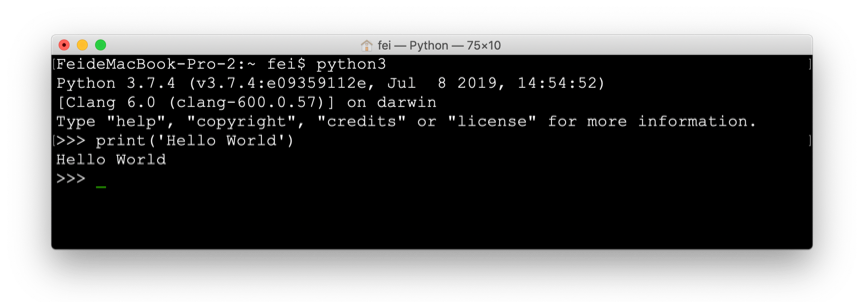4 猝不及防的Hello World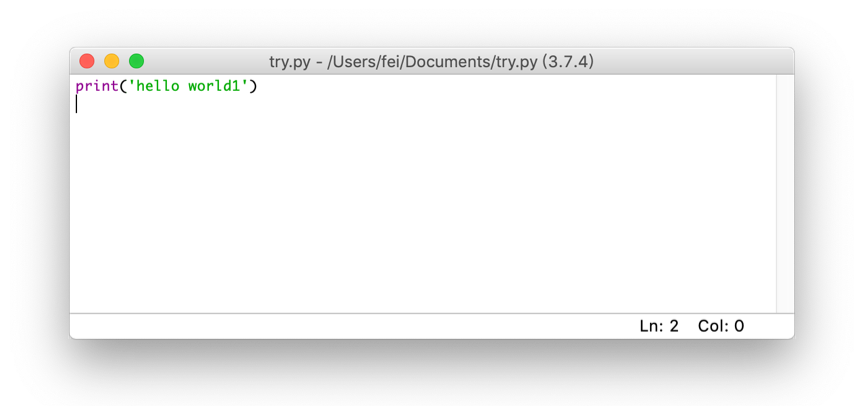5 Mac下的IDLE因为连菜单栏都分离出去了，感觉和命令行差不多了6 PyCharm 官网截图6-2 补充的一张Windows安装界面，Mac里似乎没这个问题7 PyCharm 欢迎界面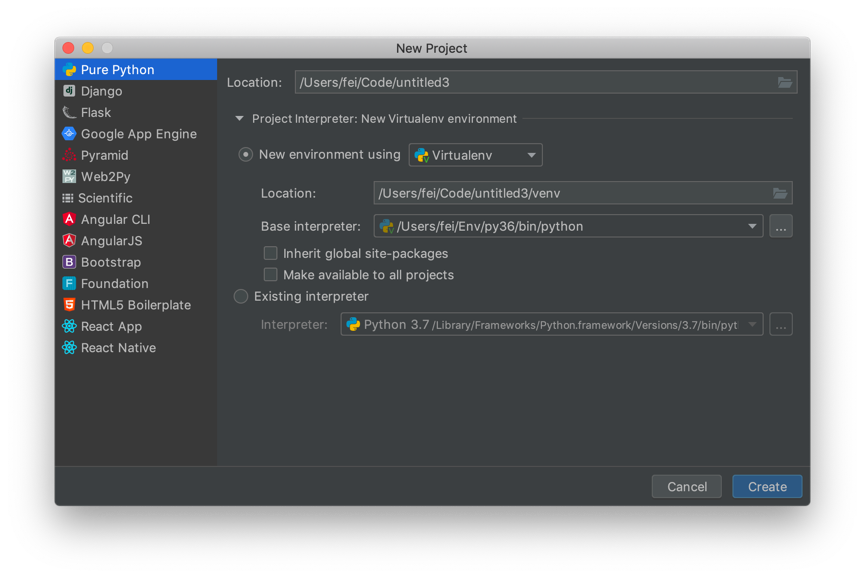8 New Project的创建页面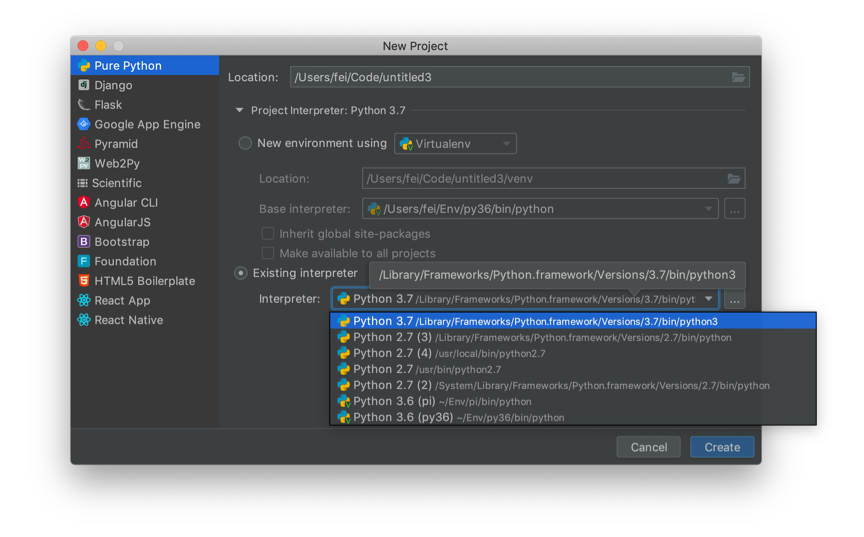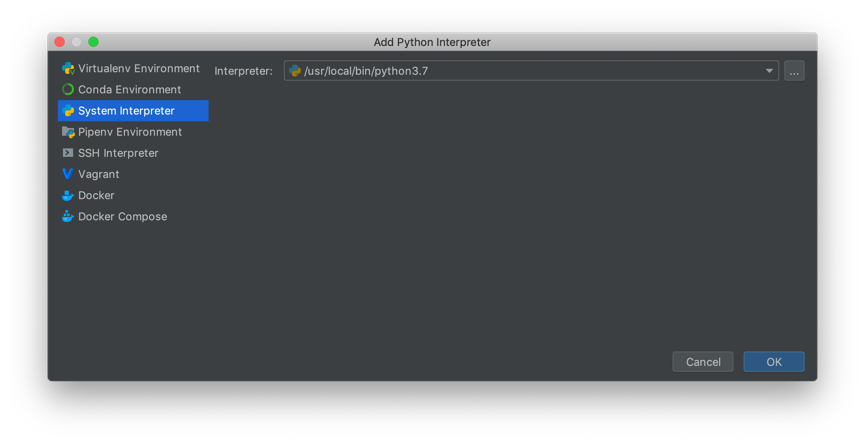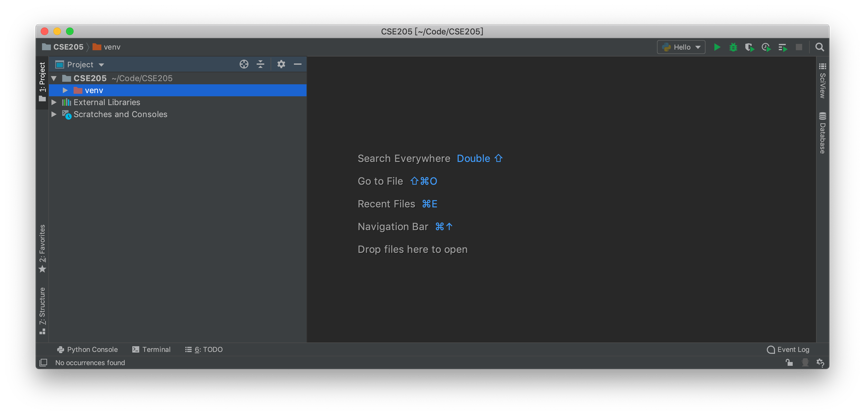11 PyCharm的工作界面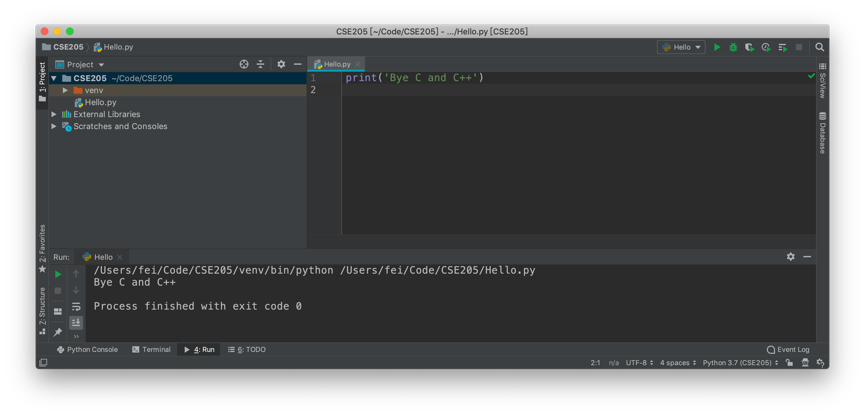12 尝试Hello World

PS: Windows如何设置环境变量：1. 按Win键，选择左边的齿轮图标——设置；
2. 搜索“环境变量”；
3. 选择“编辑系统环境变量”；
4. 在界面的右下方，选择“环境变量”；
5. 在“系统环境变量”的列表里找到 Path并双击；
6. 添加python和pip的路径。

C:\Users\xxx.xxx\AppData\Local\Programs\Python\Python37

pip的路径是：

C:\Users\xxx.xxx\AppData\Local\Programs\Python\Python37\Scripts## 解决 I2C_CheckEvent(I2C1, I2C_EVENT_MASTER_MODE_SELECT) 卡住问题和一个STM32F103的IIC示例

void IIC_Configuration()
{
// Clock Enable
RCC_APB2PeriphClockCmd(RCC_APB2Periph_AFIO | RCC_APB2Periph_GPIOB, ENABLE);
RCC_APB1PeriphClockCmd(RCC_APB1Periph_I2C1, ENABLE);
// Port Setting
GPIO_InitTypeDef  GPIO_InitStructure;
GPIO_InitStructure.GPIO_Pin = GPIO_Pin_6;
GPIO_InitStructure.GPIO_Speed = GPIO_Speed_50MHz;
GPIO_InitStructure.GPIO_Mode = GPIO_Mode_AF_OD;
GPIO_Init(GPIOB, &GPIO_InitStructure);
GPIO_InitStructure.GPIO_Pin = GPIO_Pin_7;
GPIO_Init(GPIOB, &GPIO_InitStructure);
// IIC Setting
I2C_InitTypeDef I2C_InitStruct;
I2C_InitStruct.I2C_ClockSpeed = 400000;
I2C_InitStruct.I2C_Mode = I2C_Mode_I2C;
I2C_InitStruct.I2C_DutyCycle = I2C_DutyCycle_2;
I2C_InitStruct.I2C_Ack = I2C_Ack_Enable;
I2C_Init(I2C1, &I2C_InitStruct);
// IIC Enable
I2C_Cmd(I2C1, ENABLE);
}


while(I2C_GetFlagStatus(I2C1, I2C_FLAG_BUSY));// Wait IIC Bus Idle
I2C_GenerateSTART(I2C1, ENABLE);// Send Start Flag
while(!I2C_CheckEvent(I2C1, I2C_EVENT_MASTER_MODE_SELECT));// Wait Master Mode Ready
while(!I2C_CheckEvent(I2C1, I2C_EVENT_MASTER_TRANSMITTER_MODE_SELECTED));// Wait TX Mode Ready
I2C_SendData(I2C1, 0xAA);// Send One Byte
while(!I2C_CheckEvent(I2C1, I2C_EVENT_MASTER_BYTE_TRANSMITTED));// Wait Transmittion
I2C_GenerateSTOP(I2C1, ENABLE);// Send Stop Flag


IIC_Configuration();


I2C_Cmd(I2C1, DISABLE);


uint8_t Safe_I2C_CheckEvent(I2C_TypeDef* I2Cx, uint32_t I2C_FLAG)
{
while(!I2C_CheckEvent(I2Cx, I2C_FLAG))
{
//Complete Later #1
}
return 0; //Completed Flag
}


#define Safe_I2C_CheckEvent(x,y) if (Safe_I2C_CheckEvent_Function(x,y)) return 1## 解决 IAR下的 The connected J-Link is defective 问题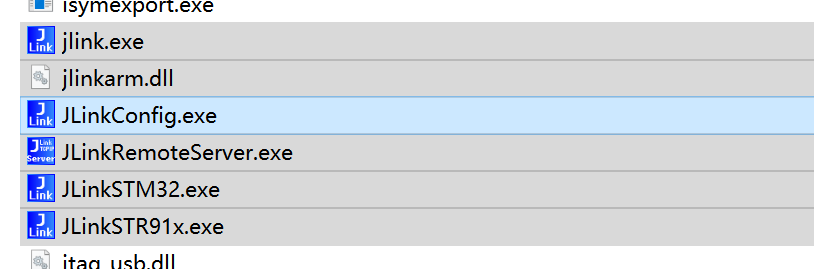# 参考文献的各种格式

## IEEE格式

IEEE的全称是电气电子工程师学会，已经成为电子信息领域统治地位的组织了。旗下所有的期刊和会议论文的引文格式均使用其自己的格式。特点是在文中用[N]来编号，在文中末尾处将所有引文按照引用的顺序排列，引文的格式遵照其规定的格式（点击查看IEEE引文规范指导）。在EEE系，使用IEEE肯定是最正确的了。不过大家并不需要记住怎么排列标题作者这些信息，Bibtex帮我们来解决。IEEE的具体形式是这样的：

This is a sentence .

 X.-C. Yin, X. Yin, K. Huang and H.-W. Hao, “Robust text detection in natural scene images,” IEEE transactions on pattern analysis and machine intelligence, vol. 36, no. 5, pp. 970-983, 2014.

## APA格式

APA是美国心理学会的简称。其特点是参考文献列表使用姓氏字母顺序排列。具体要求也很复杂（点击参考，最好别看）。其具体的形式是这样的：

This is a sentence (Yin, Yin, Huang, & Hao, 2014)

Yin, X.-C., Yin, X., Huang, K., & Hao, H.-W. (2014). Robust text detection in     natural scene images. IEEE transactions on pattern analysis and machine intelligence, 36(5), pp. 970-983.

## Chicago格式

This is a sentence (Yin, et al. 2014).

Yin, Xu-Cheng, Xuwang Yin, Kaizhu Huang, and Hong-Wei Hao. 2014. “Robust text detection in natural scene images.” IEEE transactions on pattern analysis and machine intelligence, 970-983.

## Harvard格式

Harvard格式和Chicago格式其实比较接近，只是引文列表不缩进。列表也是按照姓氏排列的。

This is a sentence (Yin, et al., 2014).

Yin, X.-C., Yin, X., Huang, K. & Hao, H.-W., 2014. Robust text detection in natural scene images. IEEE transactions on pattern analysis and machine intelligence, 36(5), pp. 970-983.

## MLA

MLA中，不在正文中出现年份，而是使用所有作者的姓氏。而且引文格式也比较简洁。

This is a sentence (Yin, Yin and Huang).

Yin, Xu-Cheng, et al. “Robust text detection in natural scene images.” IEEE transactions on pattern analysis and machine intelligence 2014: 970-983.

# LaTeX中的参考文献管理与插入

@article{yin2014robust,
title={Robust text detection in natural scene images},
author={Yin, Xu-Cheng and Yin, Xuwang and Huang, Kaizhu and Hao, Hong-Wei},
journal={IEEE transactions on pattern analysis and machine intelligence},
volume={36},
number={5},
pages={970--983},
year={2014},
publisher={IEEE}
}Google Scholar截图IEEE网站截图

\cite{yin2014robust}


\bibliographystyle{IEEEtran}
\bibliography{reference}
\addcontentsline{toc}{chapter}{Reference}# 衬线or无衬线# 等宽字体or比例字体# 如何在LaTeX里如何操作字体

## 字号（字体尺寸）

\tiny

\small

\normalsize

\large

\Large

\LARGE

\huge

\Huge

https://en.wikibooks.org/wiki/LaTeX/Fonts

https://en.wikipedia.org/wiki/Times_New_Roman# LaTeX中正常的表格绘制基本方法

\begin{table}[h]
\label{tab:tab1}
\centering
\caption{ Title Of Table }
\begin{tabular}{ |p{2cm}|p{2cm}|p{3cm}| } % #1
\hline % 绘制横向表格线，在LaTeX中需要自己定义每一行的表格线
cell 11 & cell 12 & cell 13  \\
\hline
cell 21 & cell 22 & cell 23  \\
cell 31 & cell 32 & cell 33  \\
\hline
\end{tabular}
\end{table}


p{宽度}: 指定宽度的单元格，支持多行文字自动换行，顶端对齐

m{宽度}: 指定宽度的单元格，支持多行文字自动换行，单元格垂直居中对齐

b{宽度}: 指定宽度的单元格，支持多行文字自动换行，底端对齐

l: 根据内容调整单元格宽度，不支持多行文字自动换行，左对齐

c: 根据内容调整单元格宽度，不支持多行文字自动换行，居中对齐

r: 根据内容调整单元格宽度，不支持多行文字自动换行，右对齐

，其中的1.5就是1.5倍行距，根据相应的情况改变就可以了。如果不直接指定列宽，那么LaTeX表格会根据内容进行调整宽度，并在最后留出空隙；在指定列宽的情况下，也会在文章和表格线直接留出空隙。这个空隙也是可以调整的，通过语句：\setlength{\tabcolsep}{6pt}来调整，默认就是6pt，也可以使用cm作为单位。这两条语句都是放在 table 环境里，tabular环境外。

\begin{table}[h]
\label{tab:tab1}
\centering
\caption{ Title Of Table }
\renewcommand{\arraystretch}{2}
\setlength{\tabcolsep}{10pt}
\begin{tabular}{ |>{\itshape}c|p{2cm}| >{\bfseries}m{3cm}<{\centering}| }
\hline % 绘制横向表格线，在LaTeX中需要自己定义每一行的表格线
cell 11 & cell 12 & cell 13   \\
\hline
cell 21 & cell 22 & cell 23 \\
\cline{1-2}
cell 31 & cell 32 \newline 456& cell 33  \\
\hline
\end{tabular}
\end{table}# LaTeX表格损招

\begin{table}[h]
\label{tab:tab2}
\centering
\caption{ Title Of Table }
\includegraphics[width=10cm]{fig/table.pdf}
\end{table}# 公式常用符号

## 矩阵中的省略号

https://en.wikibooks.org/wiki/LaTeX/Mathematics 这个网站讲的也非常好，就是太多了，我把有用的拿出来给大家讲讲。# 公式的一般语法

## 插入语法

1.  在行文中插入公式（inline mode）

质能方程的公式是$E=mc^2$。

2.  将公式单独显示并编号（displayed mode）

欧拉公式为：
\begin{equation}\label{equ:equation1}
e^{ix}=\cos x+i\sin x
\end{equation}

$e^{ix}=\cos x+i\sin x$

## 交叉引用

\ref{equ:xxx}

## 语法规则

\symbol{参数1}{参数2}...


# 公式的各种形式

## 上下标

A^{bc}_{de}


\lim_{x \to \infty} \exp(-x) = 0


$\lim_{x \to \infty} \exp(-x) = 0$

## 分数

\frac{分子}{分母}


z=\frac{\frac{1}{a}+\frac{1}{b}}{\frac{e}{f}-g}


^a/_b


## 根号

\sqrt[n]{m}


x=\frac{-b\pm \sqrt{b^2-4ac}}{2a}


$x=\frac{-b\pm \sqrt{b^2-4ac}}{2a}$

## 累加和积分

\sum_{n=1}^{10}\frac{1}{n}
\int_{x=1}^{10}\frac{1}{x}\,mathrm{d}x


$\sum_{n=1}^{10}\frac{1}{n}$
$\int_{x=1}^{10}\frac{1}{x}\,\mathrm{d}x$

$\sum_{\substack{ i>0 \\ j>0 }} P(i,j)$

\sum_{\substack{
i>0 \\
j>0
}}
P(i,j)


## 各种括号

( x ),[ x ],\{ x \},| x |,\| x \|,\langle x \rangle,
\lfloor x \rfloor, \lceil x \rceil, \ulcorner x \urcorner


$( x ),[ x ],\{ x \},| x |,\| x \|,\langle x \rangle, \lfloor x \rfloor, \lceil x \rceil, \ulcorner x \urcorner$

z=\left( \frac{1-x}{1+y^2} \right)


$z=\left( \frac{1-x}{1+y^2} \right)$

## 矩阵

 \begin{matrix}
a & b & c \\
d & e & f \\
g & h & i
\end{matrix}


$\begin{matrix} a & b & c \\ d & e & f \\ g & h & i \end{matrix}$

pmatrix: (), 括号

bmatrix: [], 中括号

Bmatrix: {}, 大括号

vmatrix: | |, 竖线

Vmatrix: || ||, 双竖线。

A_{m,n} =
\begin{bmatrix}
a_{1,1} & a_{1,2} & \cdots & a_{1,n} \\
a_{2,1} & a_{2,2} & \cdots & a_{2,n} \\
\vdots  & \vdots  & \ddots & \vdots  \\
a_{m,1} & a_{m,2} & \cdots & a_{m,n}
\end{pmatrix}


$A_{m,n} = \begin{bmatrix} a_{1,1} & a_{1,2} & \cdots & a_{1,n} \\ a_{2,1} & a_{2,2} & \cdots & a_{2,n} \\ \vdots & \vdots & \ddots & \vdots \\ a_{m,1} & a_{m,2} & \cdots & a_{m,n} \end{bmatrix}$

## 重音符号

https://en.wikibooks.org/wiki/LaTeX/Mathematics 这个网站讲的也非常好，就是太多了，我把有用的拿出来给大家讲讲。# 公式的一般语法

## 插入语法

1.  在行文中插入公式（inline mode）

质能方程的公式是$E=mc^2$。

2.  将公式单独显示并编号（displayed mode）

欧拉公式为：
\begin{equation}\label{equ:equation1}
e^{ix}=\cos x+i\sin x
\end{equation}

$e^{ix}=\cos x+i\sin x$

## 交叉引用

\ref{equ:xxx}

## 语法规则

\symbol{参数1}{参数2}...


# 公式的各种形式

## 上下标

A^{bc}_{de}


\lim_{x \to \infty} \exp(-x) = 0


$\lim_{x \to \infty} \exp(-x) = 0$

## 分数

\frac{分子}{分母}


z=\frac{\frac{1}{a}+\frac{1}{b}}{\frac{e}{f}-g}


^a/_b


## 根号

\sqrt[n]{m}


x=\frac{-b\pm \sqrt{b^2-4ac}}{2a}


$x=\frac{-b\pm \sqrt{b^2-4ac}}{2a}$

## 累加和积分

\sum_{n=1}^{10}\frac{1}{n}
\int_{x=1}^{10}\frac{1}{x}\,mathrm{d}x


$\sum_{n=1}^{10}\frac{1}{n}$
$\int_{x=1}^{10}\frac{1}{x}\,\mathrm{d}x$

$\sum_{\substack{ i>0 \\ j>0 }} P(i,j)$

\sum_{\substack{
i>0 \\
j>0
}}
P(i,j)


## 各种括号

( x ),[ x ],\{ x \},| x |,\| x \|,\langle x \rangle,
\lfloor x \rfloor, \lceil x \rceil, \ulcorner x \urcorner


$( x ),[ x ],\{ x \},| x |,\| x \|,\langle x \rangle, \lfloor x \rfloor, \lceil x \rceil, \ulcorner x \urcorner$

z=\left( \frac{1-x}{1+y^2} \right)


$z=\left( \frac{1-x}{1+y^2} \right)$

## 矩阵

 \begin{matrix}
a & b & c \\
d & e & f \\
g & h & i
\end{matrix}


$\begin{matrix} a & b & c \\ d & e & f \\ g & h & i \end{matrix}$

pmatrix: (), 括号

bmatrix: [], 中括号

Bmatrix: {}, 大括号

vmatrix: | |, 竖线

Vmatrix: || ||, 双竖线。

A_{m,n} =
\begin{bmatrix}
a_{1,1} & a_{1,2} & \cdots & a_{1,n} \\
a_{2,1} & a_{2,2} & \cdots & a_{2,n} \\
\vdots  & \vdots  & \ddots & \vdots  \\
a_{m,1} & a_{m,2} & \cdots & a_{m,n}
\end{pmatrix}


$A_{m,n} = \begin{bmatrix} a_{1,1} & a_{1,2} & \cdots & a_{1,n} \\ a_{2,1} & a_{2,2} & \cdots & a_{2,n} \\ \vdots & \vdots & \ddots & \vdots \\ a_{m,1} & a_{m,2} & \cdots & a_{m,n} \end{bmatrix}$

## 重音符号

https://en.wikibooks.org/wiki/LaTeX/Mathematics 这个网站讲的也非常好，就是太多了，我把有用的拿出来给大家讲讲。